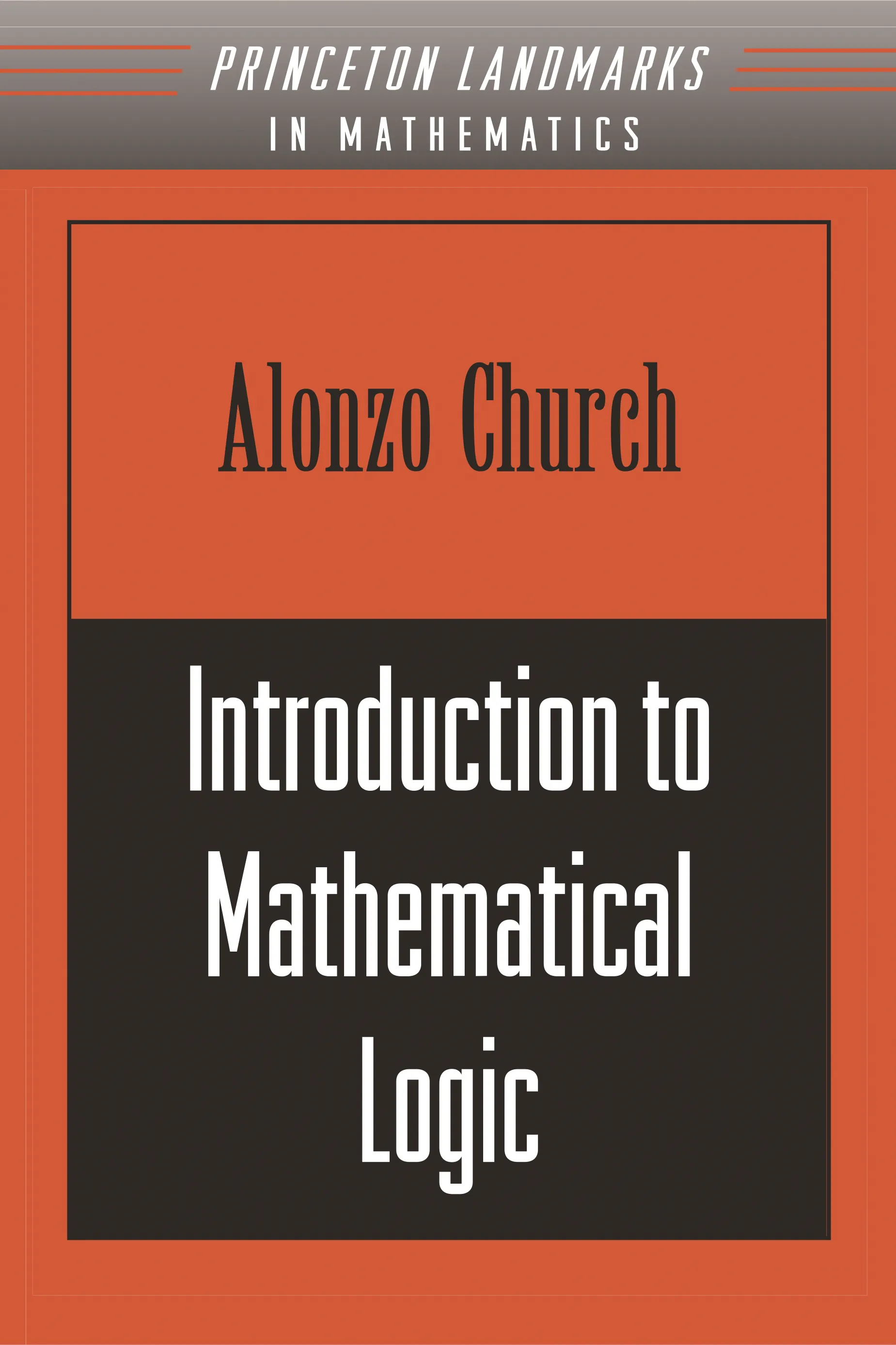Alonzo Church

# Introduction to Mathematical Logic (PMS-13), Volume 13

A classic account of mathematical logic from a pioneering giant in the field## Paperback

Price:
\$90.00/£75.00
ISBN:
Published:
Nov 17, 1996
1991
Pages:
392
Size:
6 x 9.25 in.

Logic is sometimes called the foundation of mathematics: the logician studies the kinds of reasoning used in the individual steps of a proof. Alonzo Church was a pioneer in the field of mathematical logic, whose contributions to number theory and the theories of algorithms and computability laid the theoretical foundations of computer science. His first Princeton book, The Calculi of Lambda-Conversion (1941), established an invaluable tool that computer scientists still use today.

Even beyond the accomplishment of that book, however, his second Princeton book, Introduction to Mathematical Logic, defined its subject for a generation. Originally published in Princeton’s Annals of Mathematics Studies series, this book was revised in 1956 and reprinted a third time, in 1996, in the Princeton Landmarks in Mathematics series. Although new results in mathematical logic have been developed and other textbooks have been published, it remains, sixty years later, a basic source for understanding formal logic.

Church was one of the principal founders of the Association for Symbolic Logic; he founded the Journal of Symbolic Logic in 1936 and remained an editor until 1979. At his death in 1995, Church was still regarded as the greatest mathematical logician in the world.